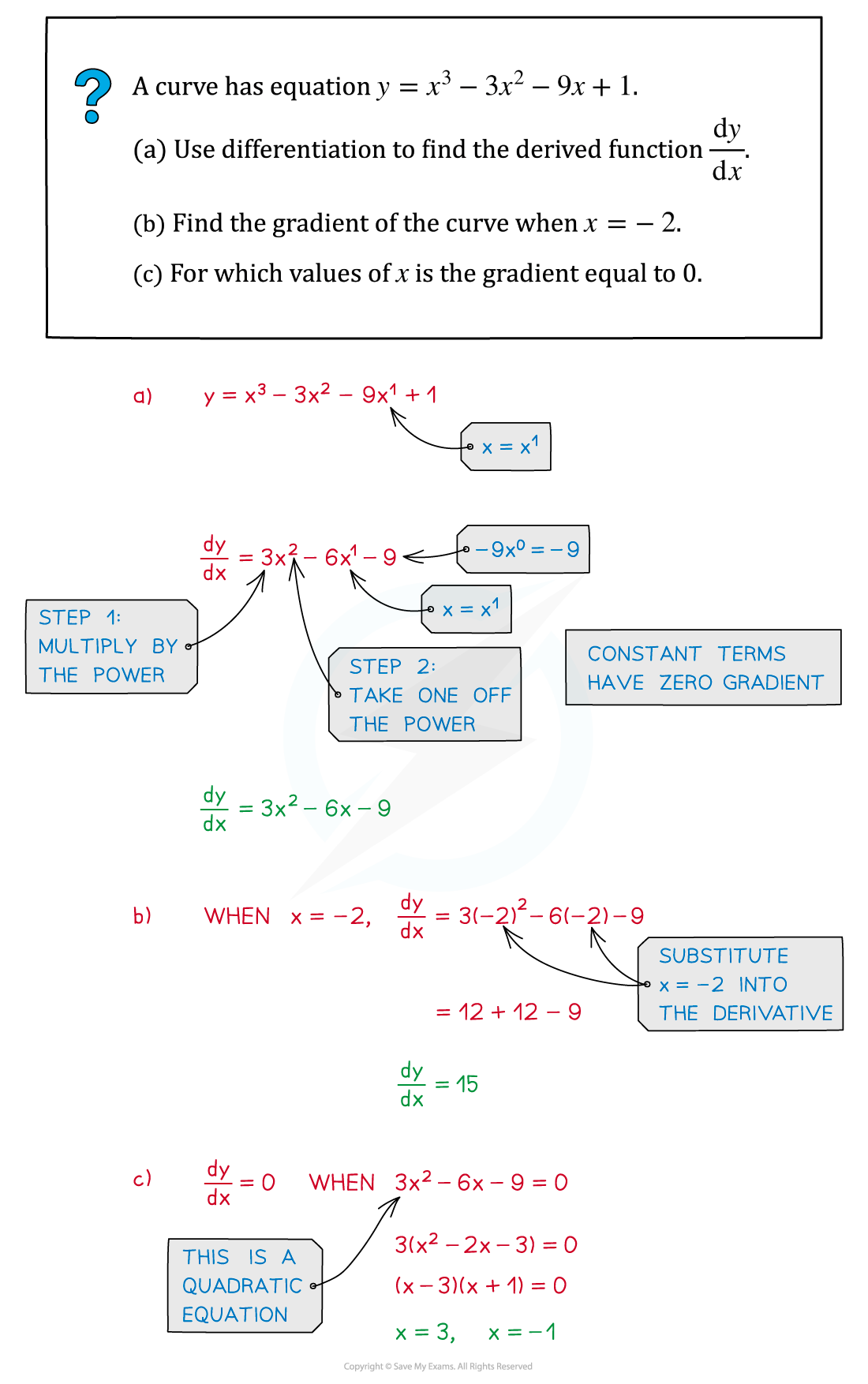# Edexcel IGCSE Maths 复习笔记 3.11.1 Differentiation - Basics

Edexcel IGCSE Maths 复习笔记 3.11.1 Differentiation - Basics

#### What is differentiation?

• Differentiation is part of the branch of mathematics called Calculus
• It is concerned with the rate at which changes takes place – so has lots of real‑world uses:
• The rate at which a car is moving – ie. its speed
• The rate at which a virus spreads amongst a population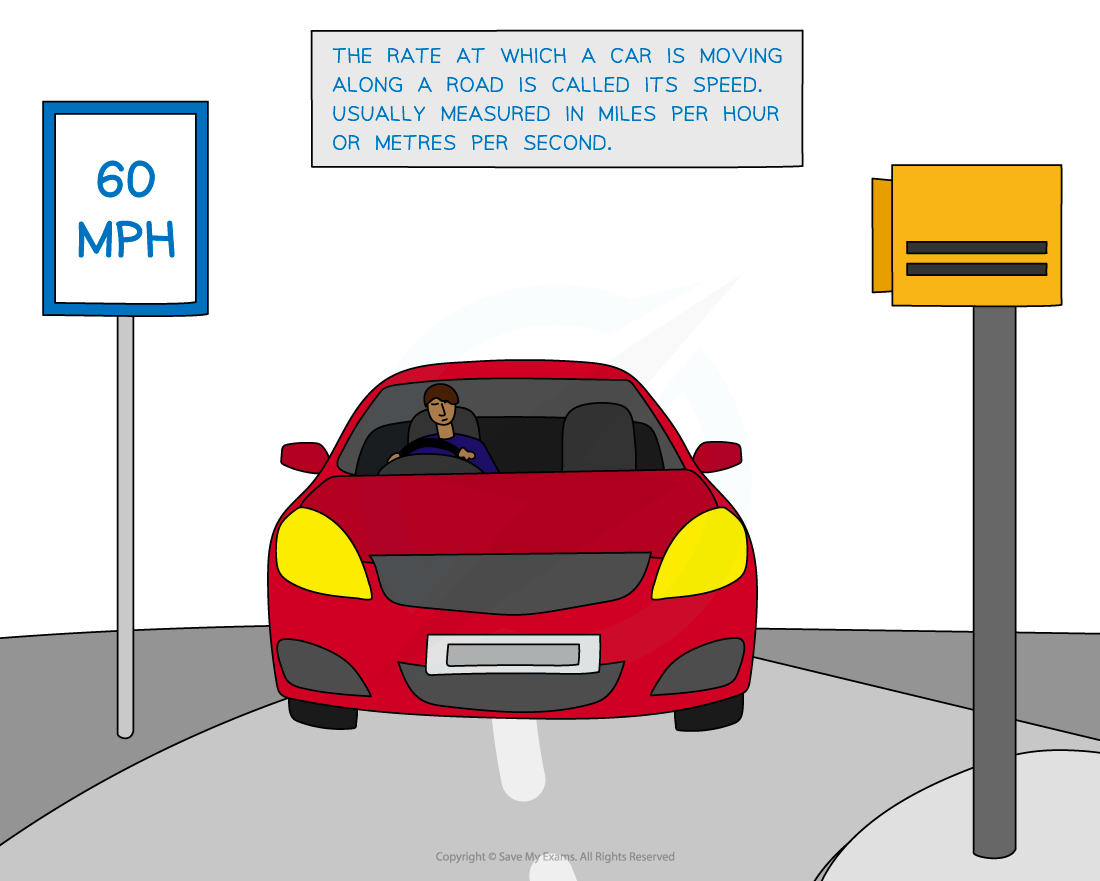• To begin to understand differentiation you’ll need to understand gradient

• For example, the gradient of a road up the side of a hill is important to lorry drivers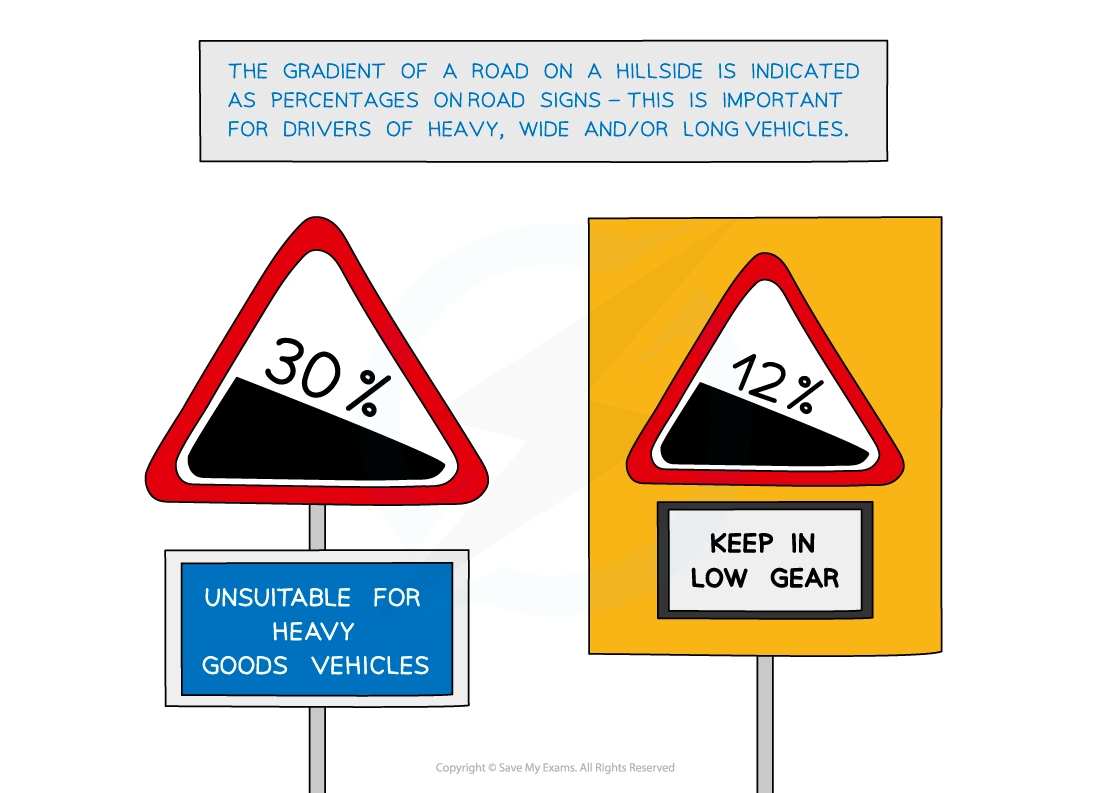• On a graph the gradient refers to how steep a line or a curve is
• It is really a way of measuring how fast y changes as x changes
• This may be referred to as the rate at which changes

• So gradient is a way of describing the rate at which change happens

#### Straight lines and curves

• For a straight line the gradient is always the same (constant)
• Recall y= mx + c, where m is the gradient (see Straight Lines - Finding Equations)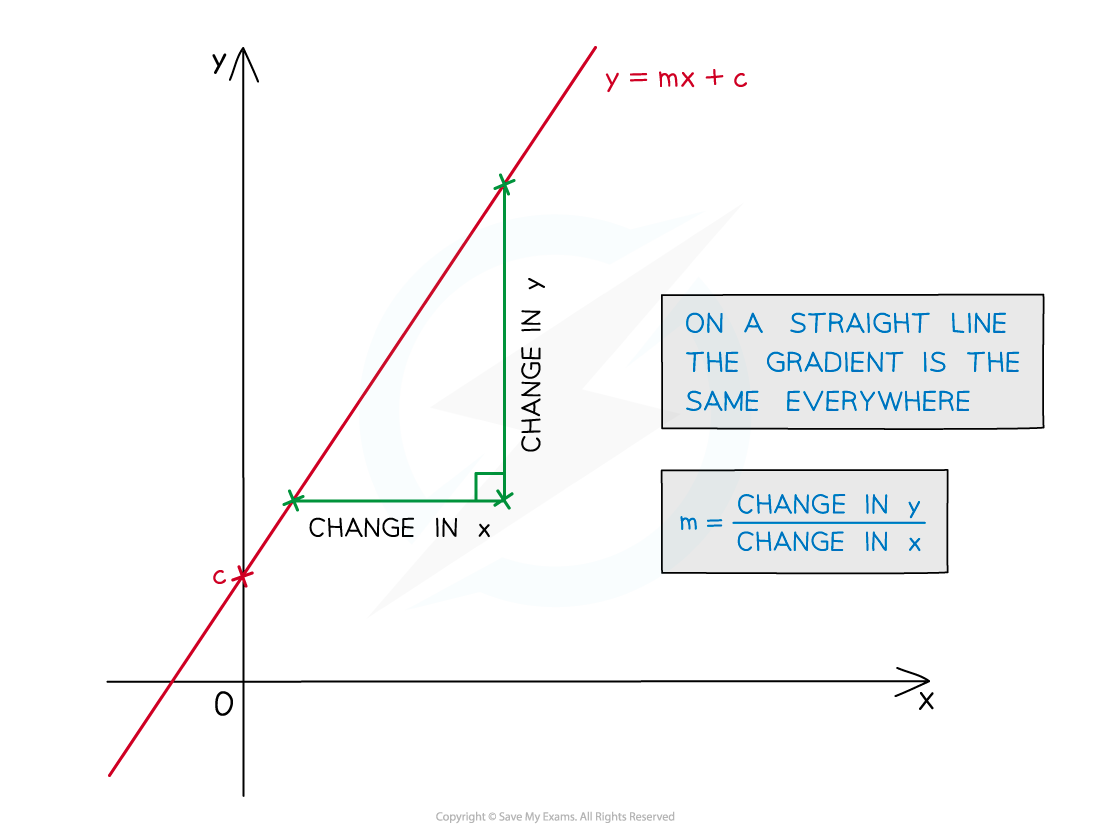• For a curve the gradient changes as the value of x changes
• At any point on the curve, the gradient of the curve is equal to the gradient of the tangent at that point
• tangent is a straight line that touches the curve at one point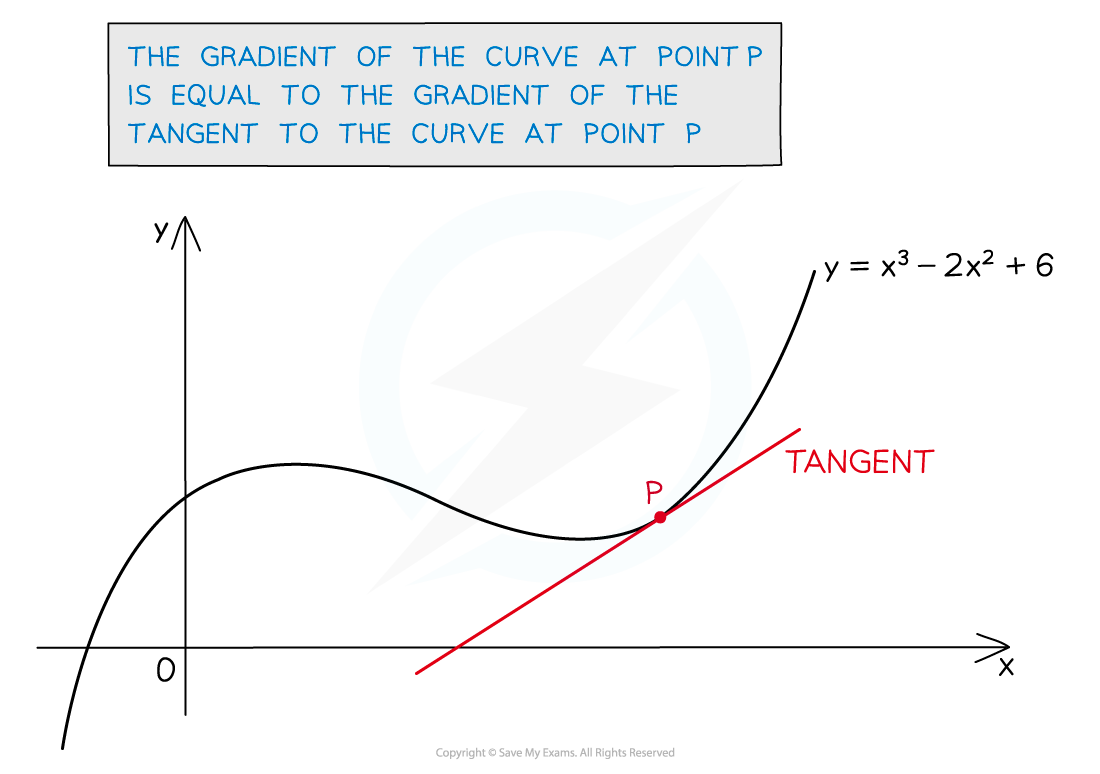• The gradient function is an expression that allows the gradient to be calculated anywhere along a curve
• The gradient function is also called the derivative

#### How do I find the gradient function or derivative?

• This is really where the fun with differentiation begins!
• The derivative (dy/dx) is found by differentiating y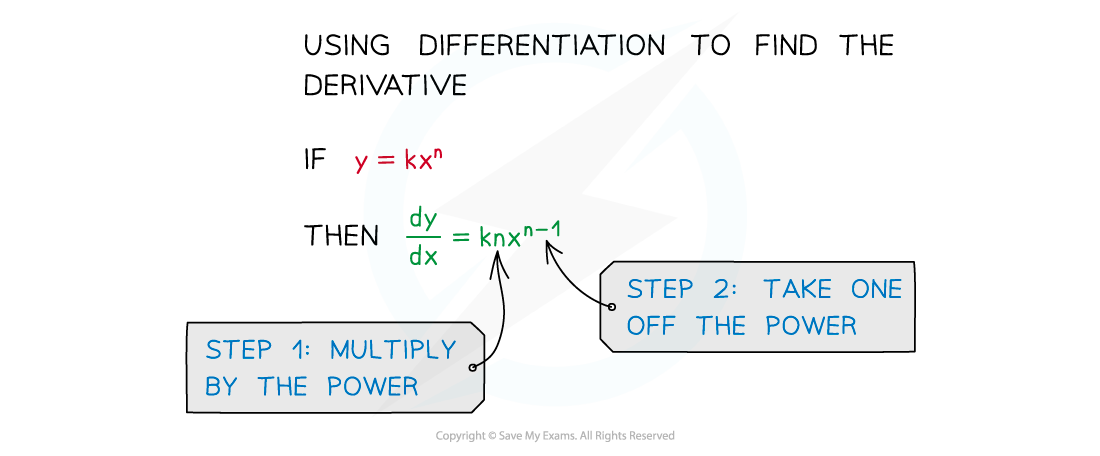• This looks worse than it is!
• For powers of x ...
• STEP 1   Multiply by the power
• STEP 2   Take one off the power• This method applies to positive and negative integers
• Negative powers arise with fractions and reciprocals#### How do I find the value of a gradient?

• Substitute the x value into the expression for the derivative, and evaluate it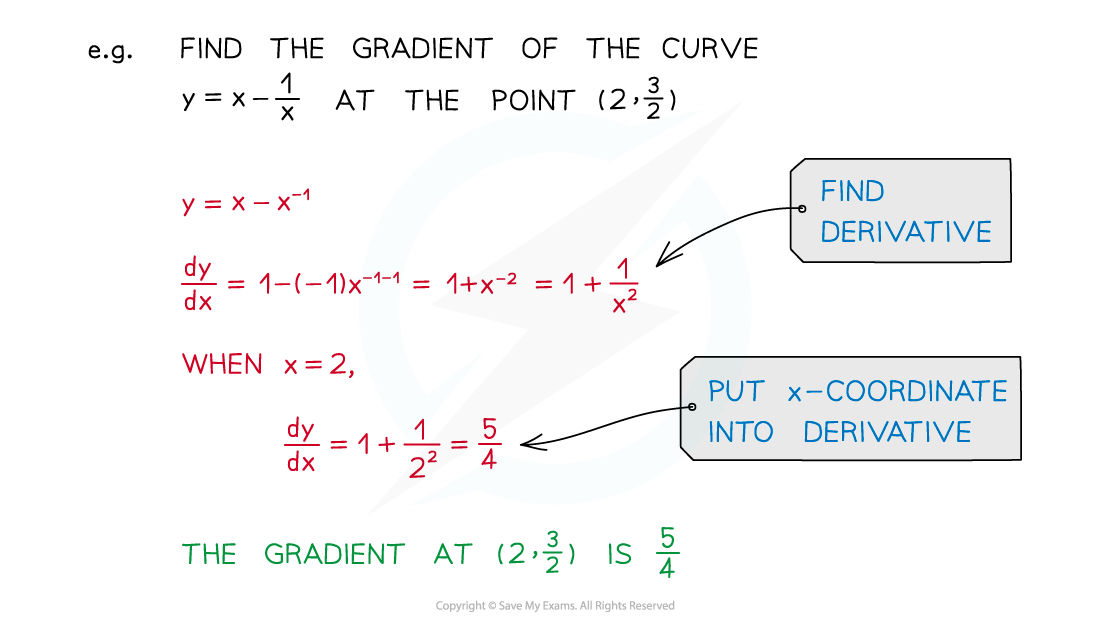#### Exam Tip

When differentiating long, awkward expressions write each step out fully and simplify afterwards.Take extra care when differentiating negative powers of x

#### Worked Example